Editor's Note: My 8th grade son, David, created a poster that he submitted to the 2013 ASA Poster Competition. The competition encourages students to display "two or more related graphics that summarize a set of data, look at the data from different points of view, and answer specific questions about the data." In this guest blog, David explains his experiment and analysis. All prize-winning posters are featured in the August 2013 issue of Amstat News.
-- RickHi! My name is David Wicklin. This blog post describes a research project and statistical graphs that I created for the 2013 ASA Poster Competition.

My poster began one day when my sister saw a small box on a store shelf that contained two spiked plastic balls. The balls were about the size of tennis balls. The box said that you should put these "dryer balls" in your dryer with a load of wet laundry. The box claimed that the balls would "reduce drying time by up to 25%." I was skeptical. Could putting plastic balls in the dryer really reduce drying time? I was looking for a project for my school science fair, so I decided to conduct an experiment to find out.

My family's dryer has a "dampness sensor" that beeps when a load of laundry is dry. I decided to use this feature and a stop watch to determine the drying time for a load of laundry. For half the loads, I'd toss in two dryer balls. For others I would not.

### Experimental Method

The first issue was how to design the experiment. One option was a controlled experiment in which I would select clothes of different materials (jeans, sweatshirts, tee shirts, and so forth) and different quantities (small, medium, and large loads). I would wash and dry each load twice: once with dryer balls and once without. That would make comparing drying times easy because the only varying factor would be the dryer balls; the clothes would be exactly the same.

However, from a practical point of view, a controlled experiment was not ideal. It would require wasting time and energy to wash and dry items twice. Plus, my family had real laundry that needed washing and drying! My dad suggested that we wash the regular family clothes and compare the mean drying times. If I washed enough laundry, I should be able to account for the random differences between the loads, such as the materials being dried. I would weigh the clothes to keep track of the size of the load.

I got my mom to agree to let me dry our family's laundry over the next few months. As you might suspect, she was very much in favor of this activity! I dried a total of 40 loads of laundry. For each load, I weighed the wet clothes by using a spring scale. For 20 randomly selected loads, I added two dryer balls to the dryer. The other 20 loads had no balls, just the clothes. Each load was dried using the same dryer setting. All types of clothing were used to randomize the samples. For each load, I recorded the weight and the drying time in a notebook.

### Analyzing and graphing the data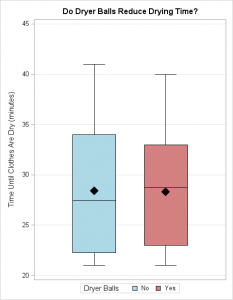I created two different graphs. The first is a side-by-side box and whisker diagram. This shows that the average drying times are not separated by much. For loads without dryer balls, the average drying time was 28.4 minutes. The median drying time was 27.5 minutes, and the first and third quartiles were 22.25 and 34 minutes. For loads with dryer balls added, the average drying time was 28.3 minutes. The median drying time was 28.75 minutes, and the first and third quartiles were 23 and 33 minutes.

The box and whisker diagram shows that it does not matter if you add dryer balls or not. If dryer balls really "reduce drying time by up to 25%," I would expect the red box plot to be shifted down by 5–8 minutes as compared to the blue box. It isn't. The two boxes are essentially the same.

But maybe dryer balls only "work" for big loads (or small loads). To find out, I created a scatter plot that displays the drying time and the weight of the wet clothes. I plotted blue squares for drying times that did not use dryer balls. I plotted red circles for times that included dryer balls.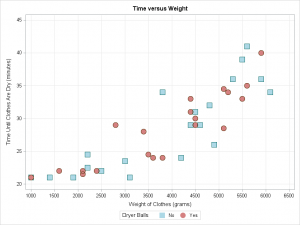The scatter plot shows that dryer balls do not affect drying time whether it is a heavy load or a light one. For light loads, it takes about 22 minutes to dry the laundry. For heavy loads it takes about 35 minutes. The red and blue markers are mixed together. If dryer balls actually reduce drying time, I would expect the red markers to be mostly below the blue markers.

The scatter plot enables me to estimate how long it will take to dry clothes, which is pretty cool! If I know that a load of laundry weighs 4 kg, I can predict that the load will take about 27 minutes to dry. I would be very confident to predict that the load will dry between 24 and 34 minutes.

The drying time obviously increases with the weight of the wet clothes. However, it is not clear if the drying time increases in a straight line or maybe a curve.

### Conclusions and Reflections

A limitation of my experiment is that I only used one washer and dryer. Our family's dryer is fairly new and our washer is "high efficiency," which means that it spins out a lot of the water. I have not done the experiment with an older, conventional, washer.

Based on my results, I conclude that the mean drying time does not depend on whether dryer balls are used. In particular, dryer balls do not “reduce drying time by up to 25%.” The observed difference was about 1%, but that could be due to randomness. The time required to dry clothes depends on the weight of the wet clothes, but using dryer balls does not make any difference in the time it takes to dry the clothes, whether the load is small or large.I am very happy to report that this poster was awarded second place in the nation for the 7–9 grade level! I am excited to be chosen for this honor. I have to thank my dad because without him I never would have been motivated enough to make this poster or record and plot 40 loads of laundry.

I got presented the award at a very special ceremony that featured the 2013 president of the ASA, Marie Davidian, a professor in the Statistics Department at NC State University. Also at the ceremony was the 2012 president, Bob Rodriguez, a senior director at SAS. They gave me a plaque and I told them all about my project. They were really nice and very friendly. It really meant a lot to me that they would take time out of their busy day to show interest in my poster and to encourage me to pursue science and math in the future.Do you have dozens (or even hundreds) of SAS data sets that you want to read into SAS/IML matrices? In a previous blog post, I showed how to iterate over a series of data sets and analyze each one. Inside the loop, I read each data set into a matrix X. I computed some quantity, and then reused X for the next data set. This technique works well, but it assumes that you only need to analyze one matrix at a time. How can you read the data sets into different matrices so that all the matrices reside in memory concurrently?

The trick is to use the SAS/IML VALSET routine to assign the contents of each data set to a unique matrix name. As I showed in a previous article, the VALSET call can dynamically create matrices whose names are specified at run time. This enables you to automate the reading of data sets into SAS/IML matrices.

The following program creates three data sets and reads the data into SAS/IML matrices whose names are identical to the data set names:

```/* create square matrices in data sets */ data A; x=1; y=2; output; x=2; y=3; output; run; data B; set A; x = x + 2; run; data C; set A; y = y + 1; run;   /* read data into SAS/IML matrices of the same name */ proc iml; dsNames = {A B C}; /* specify names of data set */ MatNames = dsNames; /* specify names of matrices */ do i = 1 to ncol(dsNames); use (dsNames[i]); read all var _NUM_ into X; /* read data into X */ call valset(MatNames[i], X); /* copy data to matrix with specified name */ close (dsNames[i]); end; free i X; show names;```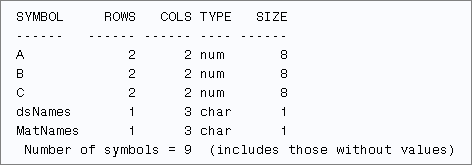The READ statement reads all numerical data into the matrix X. The VALSET call copies data from X into a matrix whose name is stored in MatNames[i]. The SHOW NAMES statement displays the names of all matrices that have values. From the output, you can see that the program creates matrices named A, B, and C.

For this example, the names of the matrices are the same as the names of the data sets. Of course, the matrices could have different names. You can assign MatName to be any array of valid names. For example, the following statement specifies the matrix names as X1, X2, and X3:

`MatNames = "x1":("x"+strip(char(ncol(dsNames))));`

You can use these ideas to read hundreds of data sets into SAS/IML matrices. For example, suppose that you need to create SAS/IML matrices for all of the numerical data in a library. You can use the DATASETS function to obtain the names of all data sets in a library. There are two special cases that you potentially need to handle:

• Some data sets might not have any numeric variables. To protect against that case, use the _ALL_ keyword to read the data. If a data set contains even one numerical variable, it will be read into the X matrix. If not, the matrix X will be a character matrix, and you can use the TYPE function to check whether X is numeric before you call the VALSET subroutine.
• Some data sets might be empty. To protect against this case, use the FREE statement to free X matrix you read each data set. If the next data set is empty, then the X matrix will be undefined. Again, the TYPE function can check for this situation.

The following SAS/IML program reads all of the data sets in the SASHELP library. On my version of SAS, there are 129 data sets in the library. Two are empty and 10 others do not contain any numerical variables. Consequently, the program defines 117 numerical matrices:

```proc iml; libref = "Sashelp"; /* read from this library */ dsNames = T( datasets(libref) ); /* 129 data sets */ MatNames = dsNames; /* names of matrices */ do i = 1 to ncol(dsNames); ds = libref + "." + strip(dsNames[i]); use (ds); /* open sashelp.filename */ read all var _ALL_ into X; /* read data into X */ if type(X)="N" then /* exclude data with zero rows or all char */ call valset(MatNames[i], X); /* copy data to matrix with specified name */ close (ds); free X; /* in case of empty data sets */ end; show names; /* 117 numerical matrices defined */```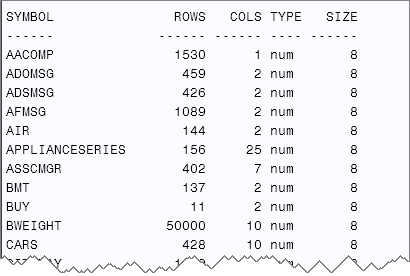The SHOW NAMES statement shows the names of a few of the matrices that were created from the numerical data in the Sashelp library. These matrices reside in memory concurrently. This technique would be useful if you have a SAS program that creates a bunch of matrices (as data sets) that you want to multiply together.

tags: Data AnalysisDo you have dozens (or even hundreds) of SAS data sets that you want to read into SAS/IML matrices? In a previous blog post, I showed how to iterate over a series of data sets and analyze each one. Inside the loop, I read each data set into a matrix X. I computed some quantity, and then reused X for the next data set. This technique works well, but it assumes that you only need to analyze one matrix at a time. How can you read the data sets into different matrices so that all the matrices reside in memory concurrently?

The trick is to use the SAS/IML VALSET routine to assign the contents of each data set to a unique matrix name. As I showed in a previous article, the VALSET call can dynamically create matrices whose names are specified at run time. This enables you to automate the reading of data sets into SAS/IML matrices.

The following program creates three data sets and reads the data into SAS/IML matrices whose names are identical to the data set names:

```/* create square matrices in data sets */ data A; x=1; y=2; output; x=2; y=3; output; run; data B; set A; x = x + 2; run; data C; set A; y = y + 1; run;   /* read data into SAS/IML matrices of the same name */ proc iml; dsNames = {A B C}; /* specify names of data set */ MatNames = dsNames; /* specify names of matrices */ do i = 1 to ncol(dsNames); use (dsNames[i]); read all var _NUM_ into X; /* read data into X */ call valset(MatNames[i], X); /* copy data to matrix with specified name */ close (dsNames[i]); end; free i X; show names;```The READ statement reads all numerical data into the matrix X. The VALSET call copies data from X into a matrix whose name is stored in MatNames[i]. The SHOW NAMES statement displays the names of all matrices that have values. From the output, you can see that the program creates matrices named A, B, and C.

For this example, the names of the matrices are the same as the names of the data sets. Of course, the matrices could have different names. You can assign MatName to be any array of valid names. For example, the following statement specifies the matrix names as X1, X2, and X3:

`MatNames = "x1":("x"+strip(char(ncol(dsNames))));`

You can use these ideas to read hundreds of data sets into SAS/IML matrices. For example, suppose that you need to create SAS/IML matrices for all of the numerical data in a library. You can use the DATASETS function to obtain the names of all data sets in a library. There are two special cases that you potentially need to handle:

• Some data sets might not have any numeric variables. To protect against that case, use the _ALL_ keyword to read the data. If a data set contains even one numerical variable, it will be read into the X matrix. If not, the matrix X will be a character matrix, and you can use the TYPE function to check whether X is numeric before you call the VALSET subroutine.
• Some data sets might be empty. To protect against this case, use the FREE statement to free X matrix you read each data set. If the next data set is empty, then the X matrix will be undefined. Again, the TYPE function can check for this situation.

The following SAS/IML program reads all of the data sets in the SASHELP library. On my version of SAS, there are 129 data sets in the library. Two are empty and 10 others do not contain any numerical variables. Consequently, the program defines 117 numerical matrices:

```proc iml; libref = "Sashelp"; /* read from this library */ dsNames = T( datasets(libref) ); /* 129 data sets */ MatNames = dsNames; /* names of matrices */ do i = 1 to ncol(dsNames); ds = libref + "." + strip(dsNames[i]); use (ds); /* open sashelp.filename */ read all var _ALL_ into X; /* read data into X */ if type(X)="N" then /* exclude data with zero rows or all char */ call valset(MatNames[i], X); /* copy data to matrix with specified name */ close (ds); free X; /* in case of empty data sets */ end; show names; /* 117 numerical matrices defined */```The SHOW NAMES statement shows the names of a few of the matrices that were created from the numerical data in the Sashelp library. These matrices reside in memory concurrently. This technique would be useful if you have a SAS program that creates a bunch of matrices (as data sets) that you want to multiply together.

tags: Data AnalysisIn a previous article I discussed how to bin univariate observations by using the BIN function, which was added to the SAS/IML language in SAS/IML 9.3. You can generalize that example and bin bivariate or multivariate data. Over two years ago I wrote a blog post on 2D binning in the SAS/IML language, but that post was written before the SAS/IML 9.3 release, so it does not take advantage of the BIN function.The image to the left shows a scatter plot of data from the Sashelp.BWeight data set. For 50,000 pregnancies, the birth weight of the baby (in grams) is plotted against the weight gain of the mother (in pounds), adjusted so that the median weight gain is centered at zero. There are 11 subintervals in the horizontal direction and seven subintervals in the vertical direction. Consequently, there are 77 cells, which you can enumerate in row-major order beginning with the upper-left cell.

A two-dimensional binning of these data enables you to find the bin for each observation. Many statistics use binned data. For example, you can do a chi-square test for association, estimate density, and test for spatial randomness. The following SAS/IML statements associates a bin number to each observation. The Bin2D function calls the BIN function twice.

```proc iml; use sashelp.bweight; read all var {m_wtgain weight} into Z; close;   /* define two-dimensional binning function */ start Bin2D(u, cutX, cutY); bX = bin(u[,1], cutX); /* bins in X direction: 1,2,...,kx */ bY = bin(u[,2], cutY); /* bins in Y direction: 1,2,...,ky */ bin = bX + (ncol(cutX)-1)*(bY-1); /* bins 1,2,...,kx*ky */ return(bin); finish;   cutX = do(-35, 75, 10); /* cut points in X direction */ cutY = do(0, 7000, 1000); /* cut points in Y direction */ b = Bin2D(Z, cutX, cutY); /* bin numbers 1-77 */```

I wrote the Bin2D function to return an index, but you can convert the index into a subscript if you prefer. (For example, the index 77 corresponds to the subscript (11, 7).) The index is useful for tabulating the number of observations in each bin. The TABULATE call counts the number of observations in each bin, which you can visualize by forming a frequency table:

```call tabulate(binNumber, Freq, b); /* count in each bin */ Count = j(ncol(cutY)-1, ncol(cutX)-1, 0); /* allocate matrix */ Count[binNumber] = Freq; /* fill nonzero counts */ print Count[c=('bX1':'bX11') r=('bY1':'bY7')];```Notice that the Y axis is reversed on the scatter plot. This makes it easy to compare the scatter plot and the tabular frequencies.

If you want to overlay the frequencies on the scatter plot, you can write the cell counts to a SAS data set and use PROC SGPLOT. The result is shown in the following figure. You can download the program used to create the figures in this blog post.

In summary, you can use the BIN function in the SAS/IML language to bin data in many dimensions. The BIN function is simpler than using the DATA step or PROC FORMAT. It handles unevenly spaced intervals and semi-infinite intervals. It's also fast. So next time you you want to group continuous data into intervals—or higher-dimensional cells!— give it a try.tags: Data AnalysisIn a previous article I discussed how to bin univariate observations by using the BIN function, which was added to the SAS/IML language in SAS/IML 9.3. You can generalize that example and bin bivariate or multivariate data. Over two years ago I wrote a blog post on 2D binning in the SAS/IML language, but that post was written before the SAS/IML 9.3 release, so it does not take advantage of the BIN function.The image to the left shows a scatter plot of data from the Sashelp.BWeight data set. For 50,000 pregnancies, the birth weight of the baby (in grams) is plotted against the weight gain of the mother (in pounds), adjusted so that the median weight gain is centered at zero. There are 11 subintervals in the horizontal direction and seven subintervals in the vertical direction. Consequently, there are 77 cells, which you can enumerate in row-major order beginning with the upper-left cell.

A two-dimensional binning of these data enables you to find the bin for each observation. Many statistics use binned data. For example, you can do a chi-square test for association, estimate density, and test for spatial randomness. The following SAS/IML statements associates a bin number to each observation. The Bin2D function calls the BIN function twice.

```proc iml; use sashelp.bweight; read all var {m_wtgain weight} into Z; close;   /* define two-dimensional binning function */ start Bin2D(u, cutX, cutY); bX = bin(u[,1], cutX); /* bins in X direction: 1,2,...,kx */ bY = bin(u[,2], cutY); /* bins in Y direction: 1,2,...,ky */ bin = bX + (ncol(cutX)-1)*(bY-1); /* bins 1,2,...,kx*ky */ return(bin); finish;   cutX = do(-35, 75, 10); /* cut points in X direction */ cutY = do(0, 7000, 1000); /* cut points in Y direction */ b = Bin2D(Z, cutX, cutY); /* bin numbers 1-77 */```

I wrote the Bin2D function to return an index, but you can convert the index into a subscript if you prefer. (For example, the index 77 corresponds to the subscript (11, 7).) The index is useful for tabulating the number of observations in each bin. The TABULATE call counts the number of observations in each bin, which you can visualize by forming a frequency table:

```call tabulate(binNumber, Freq, b); /* count in each bin */ Count = j(ncol(cutY)-1, ncol(cutX)-1, 0); /* allocate matrix */ Count[binNumber] = Freq; /* fill nonzero counts */ print Count[c=('bX1':'bX11') r=('bY1':'bY7')];```Notice that the Y axis is reversed on the scatter plot. This makes it easy to compare the scatter plot and the tabular frequencies.

If you want to overlay the frequencies on the scatter plot, you can write the cell counts to a SAS data set and use PROC SGPLOT. The result is shown in the following figure. You can download the program used to create the figures in this blog post.

In summary, you can use the BIN function in the SAS/IML language to bin data in many dimensions. The BIN function is simpler than using the DATA step or PROC FORMAT. It handles unevenly spaced intervals and semi-infinite intervals. It's also fast. So next time you you want to group continuous data into intervals—or higher-dimensional cells!— give it a try.tags: Data AnalysisIt is often useful to partition observations for a continuous variable into a small number of intervals, called bins. This familiar process occurs every time that you create a histogram, such as the one on the left. In SAS you can create this histogram by calling the UNIVARIATE procedure. Optionally, if you want to create a data set that contains the count of the observations in each bin, you can use the OUTHIST= option as shown in the following statements:

```ods select Histogram; proc univariate data=sashelp.cars; var Weight; histogram Weight / endpoints=(0 to 8000 by 1000) barlabel=count outhist=BinCount; run; /* proc print data=BinCount; run; */ /* optional print */```

The end points of the bins are called the cut points. In the histogram, the cut points are evenly spaced: 0, 1000, 2000, ..., 8000. The nine cut points define eight bins of equal width. For the Sashelp.Cars data, no vehicle weighs less than 1000 pounds, so the first bin is empty.

Two related tasks are not supported by the UNIVARIATE procedure: finding the bin number for each observation, and using unequally spaced bins. You could write a DATA step for both these tasks, but SAS/IML language provides the BIN function, which is simpler to use. (The BIN function was introduced in SAS/IML 9.3.) The following example uses the same Sashelp.Cars data:

```proc iml; use sashelp.cars; read all var {Weight} into x; close sashelp.cars;   cutpts = do(0, 8000, 1000); b = bin(x, cutpts); /* i_th element is 1-8 to indicate bin */```

The b vector indicates to which bin each observation belongs. The value of b[i] is j when x[i] is in the jth bin. If desired, you can use that information to tabulate the counts in each bin, thus creating a tabular version of the histogram:

```call tabulate(BinNumber, Freq, b); print Freq[colname=(char(BinNumber,2))];```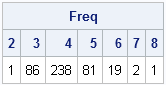The HISTOGRAM statement in PROC UNIVARIATE does not permit you to use unevenly spaced bins, but the BIN function does. In fact, the BIN function supports two special missing values. The special SAS missing value .M is interpreted as "negative infinity" and the missing value .I is interpreted as "positive infinity." An interval of the form (.M 3000) means "all observations less than 3000" and an interval of the form [4000 .I) means "all observations greater than or equal to 4000." For example, the following cut points define two semi-infinite intervals and three other unevenly spaced bins. A call to the BIN function assigns each observation to the correct bin.

```cutpts = {.M 3000 3400 3600 4000 .I}; r = bin(x, cutpts);   call tabulate(BinNumber, Freq, r); lbls = {"< 3000" "3000-3400" "3400-3600" "3600-4000" "> 4000"}; print Freq[colname=lbls];```As I have shown previously, you can use the BIN function in conjunction with the QNTL function to group observations based on quantiles.“How can we begin to make sense of the unstructured data, when we still don’t make the most of our structured data?” said the exasperated senior manager from a large retail firm. One of the great pleasures of my job is the relationship with students that continues after class has [...]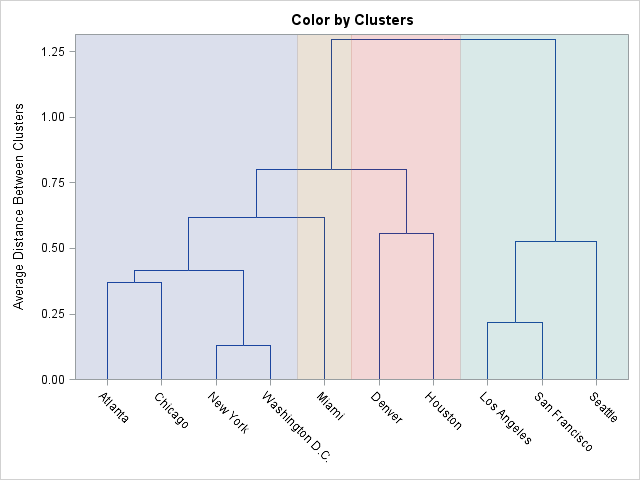The CLUSTER procedure in SAS/STAT software creates a dendogram automatically. The black-and-white dendogram is nice, but plain. A SAS customer wanted to know whether it is possible to add color to the dendogram to emphasize certain clusters. For example, the plot at the left emphasizes a four-cluster scenario for clustering cities based on the distances between cities. The question is, how can you construct such a colored dendogram?

One solution, which was proposed by my colleague Warren Kuhfeld, is to use the output of the PROC CLUSTER procedure in conjunction with the Graphics Template Language (GTL) to overlay a dendogram and a block plot. A block plot is simply a series of colored strips that span the length of a graph. You can create a block plot by using the GTL BLOCKPLOT statement.

To demonstrate how to construct a basic version of the colored dendogram, let's start with the output from the CLUSTER procedure when applied to the Sashelp.Mileages data set, which contains distances between 10 major US cities:

```proc cluster data=Sashelp.Mileages(type=distance) method=average pseudo out=Tree; id City; run;```

From the procedure output, you can see that the City variable is used to construct the horizontal axis. To overlay a block plot, you can create a simple data set that identifies each city with a colored block. The following DATA step specifies the cities in alphabetical order, along with their "cluster number." This information is merged with a sorted version of the Tree data set. The Tree2 data set contains the information needed to construct the colored dendogram:

```data Clusters; input City \$ 1-15 Cluster; datalines; Atlanta 1 Chicago 1 Denver 3 Houston 3 Los Angeles 4 Miami 2 New York 1 San Francisco 4 Seattle 4 Washington D.C. 1 ;   proc sort data=tree; by city; run; data tree2; merge Tree Clusters; by City; run;```

Now that the data are properly prepared, you can define a GTL template that overlays a dendogram and a block plot. The SGRENDER procedure is used to create the graph that is shown at the beginning of this article:

```proc template; define statgraph Dendrogram; begingraph; entrytitle "Color by Clusters"; layout overlay / yaxisopts=(discreteopts=(tickvaluefitpolicy=none)); dendrogram nodeid=_name_ parentid=_parent_ clusterheight=_height_; blockplot x=City block=Cluster / datatransparency=0.75 display=(fill); endlayout; endgraph; end; run;   proc sgrender data=tree2 template=dendrogram; run;```

This graphical technique enables you to emphasize the grouping of the cities into four clusters. If you want to emphasize a different number of clusters, you need to re-create the Clusters data set, re-merge the data, and call PROC SGRENDER again. This can be tedious, so Warren Kuhfeld wrote a macro that automates this process. The result is the %ClusterGroups macro, which is available for download from support.sas.com. The macro enables you to create colored dendograms easily. For example, after running the CLUSTER procedure, the following statement creates the colored dendogram in this article:

`%clustergroups(nclusters=4, data=Tree, id=City)`

The macro takes care of generating the auxiliary data set, merging it with the output from PROC CLUSTER, writing the GTL template, and calling PROC SGRENDER. You can specify the number of clusters that you want to emphasize, and it computes the appropriate range for the colored blocks.

Give it a try and let me know what you think. I'll pass on your comments to Warren Kuhfeld, who deserves all the credit.The CLUSTER procedure in SAS/STAT software creates a dendrogram automatically. The black-and-white dendrogram is nice, but plain. A SAS customer wanted to know whether it is possible to add color to the dendrogram to emphasize certain clusters. For example, the plot at the left emphasizes a four-cluster scenario for clustering cities based on the distances between cities. The question is, how can you construct such a colored dendrogram?

One solution, which was proposed by my colleague Warren Kuhfeld, is to use the output of the PROC CLUSTER procedure in conjunction with the Graphics Template Language (GTL) to overlay a dendrogram and a block plot. A block plot is simply a series of colored strips that span the length of a graph. You can create a block plot by using the GTL BLOCKPLOT statement.

To demonstrate how to construct a basic version of the colored dendrogram, let's start with the output from the CLUSTER procedure when applied to the Sashelp.Mileages data set, which contains distances between 10 major US cities:

```proc cluster data=Sashelp.Mileages(type=distance) method=average pseudo out=Tree; id City; run;```

From the procedure output, you can see that the City variable is used to construct the horizontal axis. To overlay a block plot, you can create a simple data set that identifies each city with a colored block. The following DATA step specifies the cities in alphabetical order, along with their "cluster number." This information is merged with a sorted version of the Tree data set. The Tree2 data set contains the information needed to construct the colored dendrogram:

```data Clusters; input City \$ 1-15 Cluster; datalines; Atlanta 1 Chicago 1 Denver 3 Houston 3 Los Angeles 4 Miami 2 New York 1 San Francisco 4 Seattle 4 Washington D.C. 1 ;   proc sort data=tree; by city; run; data tree2; merge Tree Clusters; by City; run;```

Now that the data are properly prepared, you can define a GTL template that overlays a dendrogram and a block plot. The SGRENDER procedure is used to create the graph that is shown at the beginning of this article:

```proc template; define statgraph Dendrogram; begingraph; entrytitle "Color by Clusters"; layout overlay / yaxisopts=(discreteopts=(tickvaluefitpolicy=none)); dendrogram nodeid=_name_ parentid=_parent_ clusterheight=_height_; blockplot x=City block=Cluster / datatransparency=0.75 display=(fill); endlayout; endgraph; end; run;   proc sgrender data=tree2 template=dendrogram; run;```

This graphical technique enables you to emphasize the grouping of the cities into four clusters. If you want to emphasize a different number of clusters, you need to re-create the Clusters data set, re-merge the data, and call PROC SGRENDER again. This can be tedious, so Warren Kuhfeld wrote a macro that automates this process. The result is the %ClusterGroups macro, which is available for download from support.sas.com. The macro enables you to create colored dendrograms easily. For example, after running the CLUSTER procedure, the following statement creates the colored dendrogram in this article:

`%clustergroups(nclusters=4, data=Tree, id=City)`

The macro takes care of generating the auxiliary data set, merging it with the output from PROC CLUSTER, writing the GTL template, and calling PROC SGRENDER. You can specify the number of clusters that you want to emphasize, and it computes the appropriate range for the colored blocks.

Give it a try and let me know what you think. I'll pass on your comments to Warren Kuhfeld, who deserves all the credit.A regular reader noticed my post on initializing vectors by using repetition factors and asked whether that technique would be useful to expand data that are given in value-frequency pairs. The short answer is "no." Repetition factors are useful for defining (static) matrix literals. However, if you want to expand data dynamically, I recommend that you use the REPEAT function or the technique in the article on expanding data by using frequencies.

For example, the following SAS/IML statement defines a vector for which the value 2.2 is repeated two times and the value 3.3 is repeated three times. The resulting vector has five elements:

```proc iml; x = { 2.2  3.3};```

This vector is constructed as a matrix literal. If instead you have the values and frequencies in separate vectors, then use the ExpandFreq module in my previous post:

```values = {2.2 3.3}; freq = {2 3}; load module=(ExpandFreq); /* define or load ExpandFreg module here */ y = ExpandFreq(values, freq);```

This is probably a good time to remind everyone about the SAS/IML Support Community. You can post your SAS/IML questions there 24 hours a day. That is always better than sending me direct email. There are lots of experienced SAS/IML experts out there, so use the SAS/IML Support Community to tap into that knowledge.

tags: Data Analysis